# Easier to recursively build than recursively deconstruct... is it even doable? (yes!)

## Recommended PostsNot the 1rst time I try this, but I always managed to find another route. This time I don't want any "hard coded alternative" so...please bare with me. I'm going from a list like that which corresponds to the selection portion of ssnamex  (so its length can vary)

```(setq Lst
((-4 (0 (269.157 85.9418 0.0)) (0 (272.343 85.9418 0.0)) (0 (272.343 59.9463 0.0)) (0 (269.157 59.9463 0.0)))
(-3 (0 (273.021 84.9263 0.0)) (0 (274.851 84.9263 0.0)) (0 (274.851 83.3016 0.0)) (0 (273.021 83.3016 0.0)))
(-2 (0 (275.258 65.4297 0.0)) (0 (279.528 65.4297 0.0)) (0 (279.528 60.6233 0.0)) (0 (275.258 60.6233 0.0)))
(-1 (0 (280.206 72.2671 0.0)) (0 (283.596 72.2671 0.0)) (0 (283.596 69.5592 0.0)) (0 (280.206 69.5592 0.0)))
)
)```

Since the length of the list can vary, I thought "recursion", but fast found that while recursive building is somewhat of an easy task, recursively deconstruct seems quite another story. (can it be done?)
So far my best attempt manage to remove everything that is not a coord, but the list structure is the same as the original list, like that

```;Not quite there yet!
(defun keepcoords (L)
(if (listp L)
(if (and (atom (car L))
)
L
(mapcar '(lambda (x)
(keepcoords x)
)
L
)
)
)
)
;returns that....
((nil (nil (269.157 85.9418 0.0)) (nil (272.343 85.9418 0.0)) (nil (272.343 59.9463 0.0)) (nil (269.157 59.9463 0.0)))
(nil (nil (273.021 84.9263 0.0)) (nil (274.851 84.9263 0.0)) (nil (274.851 83.3016 0.0)) (nil (273.021 83.3016 0.0)))
(nil (nil (275.258 65.4297 0.0)) (nil (279.528 65.4297 0.0)) (nil (279.528 60.6233 0.0)) (nil (275.258 60.6233 0.0)))
(nil (nil (280.206 72.2671 0.0)) (nil (283.596 72.2671 0.0)) (nil (283.596 69.5592 0.0)) (nil (280.206 69.5592 0.0))))```

What I need is to end up with a list containing only the coords, like that

`((269.157 85.9418 0.0)(272.343 85.9418 0.0)(272.343 59.9463 0.0)(269.157 59.9463 0.0)(273.021 84.9263 0.0)...and so on...)`

I know that I could "hardcode" a solution that would work for (and only for) that specific type of list, but my goal here is to make a function that could work to extract only the point coords of lists of any length and morphology, and recursion seems the only way it could be achieved. My poor brain is now broken. I need some new perspective on that one. Please unbreak my brain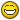##### Share on other sitesAh, I might have forgotten to mention other arbitrary decided criteria, such as "only 1 function" and "no subfunctions".. because

```(defun keepcoords ( L / helper ret)
(defun helper ( L )
(if (listp L)
(if (and (atom (car L))
(numberp (car L))
(= 3 (length L))
)
(setq ret (cons L ret));L
(mapcar '(lambda (x)
(helper x)
)
L
)
)
)
)
(helper L)
ret
)```

seems less elegant than a simple building recursion. But it works. Still, feel free to post if you can find a way to achieve it in a single function.

Edited by Jef!
•1

##### Share on other sitesSomething like this perhaps?

```(defun coords ( lst )
(if (and lst (listp lst))
(if (and (< 1 (length lst) 4) (vl-every 'numberp lst))
(list lst)
(append (coords (car lst)) (coords (cdr lst)))
)
)
)```

•2

##### Share on other sites50 minutes ago, Lee Mac said:

Something like this perhaps?

```
(defun coords ( lst )
(if (and lst (listp lst))
(if (and (< 1 (length lst) 4) (vl-every 'numberp lst))
(list lst)
(append (coords (car lst)) (coords (cdr lst)))
)
)
)﻿```

Ahhh! YES! One of my tries was so close to that, but instead of keeping coords in list just flattened them to a single list because I returned the lst instead of (list lst). I so always avoid append as often as I can that apparently I don't even know how to use it properly. Here'S what I had

```;one of my failures
(defun keepcoords (L)
;Converts to single level...:(
(if (listp L)
(if (and (atom (car L))
)
L
(append (keepcoords (car L)) (keepcoords (cdr L))))
nil))
;returns (269.157 85.9418 0.0 272.343 85.9418 0.0 272.343 59.9463 0.0 269.157 59.9463 0.0 .....```

It is crazy what we see once someone opens our eyes. Your "(and lst (listp lst))" is a good reminder that (listp nil) returns T, which explain why I had some internal stack limit issues trying to modify my append version, before I went crazy-berserk using nested ifs, mapcars, lambdas, a subfunction and a local variable. Like the proverb says: "We live, read Lee Mac and learn."

Thank you Lee!

edit: hats off for the (< 1 (length lst) 4)&(vl-every 'numberp lst) as well. More elegant than what I did.

Edited by Jef!

##### Share on other sites```(defun foo ( L / pointp r)
(defun pointp ( p ) (and (vl-consp p) (= 3 (length p)) (apply 'vl-every (cons 'numberp (list p)))))
(while L
(setq r (append (vl-remove-if-not 'pointp L) r))
(setq L (apply 'append (vl-remove-if-not 'vl-consp L)))
); while
r
); defun foo```
```_\$ (equal (coords lst) (foo lst) 1e-2)
T```

59 minutes ago, Jef! said:

(and lst (listp lst))

The above check equals to (vl-consp lst)

```(defun f ( L )
(cond
( (atom L) nil)
( (append (f (car L)) (f (cdr L))) )
( (and (= 3 (length L)) (vl-every 'numberp L)) (list L) )
)
)```

Edited by Grrr
recursive syntax that might be understood by jef!
•2

##### Share on other sitesAnother

```(defun coords (lst)
(apply 'append
(mapcar
'(lambda (x)
(cond
((atom x) nil)
((vl-every 'numberp x) (list x))
((coords x))
)
)
lst
)
)
)```
•2

##### Share on other sites8 minutes ago, Stefan BMR said:

Another

I like yours, Stefan.. but I would replace this part

```(mapcar
...
lst
)```

like so

```(mapcar
...
(cond ((listp lst) lst))
)
```

so it won't break on stuff like

`(coords 1)`

But I agree that my nitpick is an individual opinion to one, since some subfoos are still accepted without self-input-checking.

##### Share on other sitesonly applicable for your list structure - without recursive

```
(apply 'append (mapcar ''((x)(mapcar 'cadr (cdr x))) lst ))```

•1

##### Share on other sites17 minutes ago, Grrr said:

I like yours, Stefan.. but I would replace this part

```
(mapcar
...
lst
)```

like so

```
(mapcar
...
(cond ((listp lst) lst))
)
```

so it won't break on stuff like

```
(coords 1)```

But I agree that my nitpick is an individual opinion to one, since some subfoos are still accepted without self-input-checking.

Yes, (coords 1) will error... But also, every single construct like (mapcar ... lst) will fail if lst is an atom. Are you checking this EVERY time? What about other functions? Do + function need checking for EVERY argument, EVERY time you use it?

##### Share on other sites4 minutes ago, Stefan BMR said:

But also, every single construct like (mapcar ... lst) will fail if lst is an atom. Are you checking this EVERY time? What about other functions? Do + function need checking for EVERY argument, EVERY time you use it?

I do this only on my subfunctions, esp the large ones - and not on the built-in lisp functions. Like I said its an individual opinion, that slows down the whole routine and its drastically slowed when the subfoo is recursive.

##### Share on other sites7 minutes ago, Grrr said:

I do this only on my subfunctions, esp the large ones - and not on the built-in lisp functions. Like I said its an individual opinion, that slows down the whole routine and its drastically slowed when the subfoo is recursive.

Fair enough. For debug reasons, I don't check this kind of possible errors. And, of course, I don't add it once the routine is bug free##### Share on other sitesFWIW Just a compromise approach to check the initial input (provided by the subfoo's user) is to transform the recursion into a tail one:

```(defun _coords ( lst )
(
(lambda (coords)
(if (vl-consp lst) (coords lst)) ; <- initial check, if ok start recursin'
)
(lambda (lst) ; coords, which was (defun coords (lst) ...)
(apply 'append
(mapcar
'(lambda (x)
(cond
((atom x) nil)
((vl-every 'numberp x) (list x))
((coords x))
)
)
lst
)
)
)
)
); defun```

Sorry for the offtopic I caused.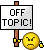##### Share on other sites20 hours ago, Grrr said:

so it won't break on stuff like

20 hours ago, Stefan BMR said:

Yes, (coords 1) will error... But also, every single construct like (mapcar ... lst) will fail if lst is an atom. Are you checking this EVERY time? What about other functions? Do + function need checking for EVERY argument, EVERY time you use it?

That is a very interesting topic altogether. Sometimes I go out of my way to validate input and trap errors inside subfunctions, and can become quickly quite complicated. Is it the subfunction's role to validate the input provided by the "parent function", or is it better to have the parent function ensure that it calls the correct sub function along with valid arguments? For sure some validations have to be made somewhere, but where is optimal/better? I'm not sure there is an universal answer to that. (Without checking, I think most of the time I do error traping on the parent side, and validation inside the children starting with an if or an and...) To think of it, for a recursive function, tho, it has to validate before it relaunches itself, so it has to do it anyway. If it does it down the line to determine if it should relaunch itself (validation on the "parent side"), it still won'T prevent the initial launch to bomb. On the other hand, if it validates first (validation on the children side), and then throw everything left recursively regardless, it looks safer as any initial input will get validated too.

20 hours ago, hanhphuc said:

only applicable for your list structure - without recursive

```
(apply 'append (mapcar ''((x)(mapcar 'cadr (cdr x))) lst ))﻿```

Even if that one is only applicable for that specific list structure, I have to bow down to the ingenuity of the double quotes that I cannot fully understand and wrap my head around... How did you come up with that? (of course since it is not recursive its speed is incredible. Even if comparison is uneven, for the fun of comparing apples and bananas...Quote

Elapsed milliseconds / relative speed for 16384 iteration(s):
(QUOTE (APPLY (QUOTE APPEND) (MAPCAR...)......1310 / 23.52 <fastest>
(LMCOORDS L)..................................5601 / 5.50
(GRRRFOO2 L)..................................6240 / 4.94
(BMRCOORDS L).................................8003 / 3.85
(GRRRFOO L)..................................30077 / 1.02
(KEEPCOORDS L)...............................30810 / 1.00 <slowest>

I'm lumping behind. hahahaha. Thanks for the enlightenment.
(and now the cake distribution starts!)

edit2: @Grrr: I really like your f (the cond version) you made. Simple, clean/easy to read and fast.

Edited by Jef!
god dammmned html highlighting!!! ^^

##### Share on other sites2 hours ago, Jef! said:

Is it the subfunction's role to validate the input provided by the "parent function", or is it better to have the parent function ensure that it calls the correct sub function along with valid arguments?

I've forgot to elaborate that I always do input checking on my larger subfunctions -
Because the last thing I would like to do again is to waste time on debugging a 300 row subfoo, when the fault is in my main that provided the wrong inputs.
So a simple structure using cond that originated from Lee's DCL on-the-fly is enough for me:

```; Sample subfoo of 100+ rows (cutted)
(defun PDF->JPG ( qlty Lfpaths / Trap *error* psAPP jpegSaveOptions PDFOpenOptions r psDoc psDocs )
(cond
( (not (<= 0 qlty 12)) )
( (not Lfpaths) (prompt "\nNo list of filepaths specified.") )
( (not (setq psAPP (vlax-get-or-create-object "Photoshop.Application"))) (prompt "\nUnable to interface with Photoshop Application.") )
( (not (setq jpegSaveOptions (vlax-get-or-create-object "Photoshop.JPEGSaveOptions"))) (prompt "\nUnable to interface with JPEGSaveOptions.")  )
( (not (setq PDFOpenOptions (vlax-get-or-create-object "Photoshop.PDFOpenOptions"))) (prompt "\nUnable to interface with PDFOpenOptions.")  )
( ...do my stuff... )
); cond
); defun ```

Ofcourse I also write shorties that do not check inputs, but usually they are lambdas inside of my main.

In order not to be misunderstood I'll say it again: thats my personal preference.

2 hours ago, Jef! said:

edit2: @Grrr: I really like your f (the cond version) you made. Simple, clean/easy to read and fast.﻿

Thanks, I won't forget Michael Puckett's keywords on when I started practicing linear recursions

Quote

MP
Hint: cond, null, atom.

So till then, for me the cond functions creates the most understandable version of a given recursion.

Still Lee Mac comes up with some insane recursive solutions upon my old requests that I still can't get over my head. Like map the mapcar.. its just mind blowing.

Cheers!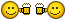##### Share on other sites2 hours ago, Grrr said:

Thanks, I won't forget Michael Puckett's keywords on when I started practicing linear recursions

Quote

MP
Hint: cond, null, atom.

I'm not sure what MP's background is, but he seems very, very skilled. Kind of things that are not in Adesk online help...^^ Makes a lot of sense. What I did is to only react to lists, check if it contained coords, if not recurse each list elements individually, in other words null triggers nothing.
Fun fact: I was totally blown away by hanhphuc 's double single quotes, and in the topic you just linked you had one. I like Gile's understanding level of these complex concepts in the following post and how he "deconstruct" them. "x is the same as this which are both equal and evaluate like that". I will have to read that next week. Might help me understand what hanhphuc did##### Share on other sites7 hours ago, Jef! said:

I'm not sure what MP's background is, but he seems very, very skilled

Alot, somewhere he told me when he started lisping (a few decades before I was born)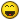initially I underestimated him because of this subfoo, I questioned myself "why the heck someone will copyright a simple function like this".

But after that I've found his help/work on the forum that he's active and I realised how wrong my impression was.

7 hours ago, Jef! said:

Fun fact: I was totally blown away by hanhphuc 's double single quotes, and in the topic you just linked you had one.

I saw that technique from hanhphuc too (because he uses it too often, and sometimes rarely from Lee).

And its not that hard to understand it -

knowing that '((x)(* (+ x 1) 2)) equals to (lambda(x)(* (+ x 1) 2)) , so a single quote substitutes the lambda function

```'((x)(* (+ x 1) 2))
(lambda(x)(* (+ x 1) 2))```

then you can compare, by running with some inputs:

```(
'((x)(* (+ x 1) 2))
3
)
>> 8

(
(lambda(x)(* (+ x 1) 2))
3
)
>> 8```

so when you intend to map that function with mapcar, then you'll need the additional apostrophe for both

```_\$ (mapcar ''((x)(* (+ x 1) 2)) '(1 2 3))
>> (4 6 8)
_\$ (mapcar '(lambda(x)(* (+ x 1) 2)) '(1 2 3))
>> (4 6 8)```

however you can't do this:

```(mapcar (function '((x)(* (+ x 1) 2))) '(1 2 3))
bad FUNCTION argument: (QUOTE ((X) (* (+ X 1) 2)))```

Since compiling to vlx requires using the function function and not an apostrophe, means that you won't be able to convert this syntax to a .vlx file

In summary this type of function is anonymous and it has a LIST type:

```_\$ (type '((x)(* (+ x 1) 2)))
LIST
_\$ (type (lambda(x)(* (+ x 1) 2)))
USUBR```

IMO since its treated as unevaluated list I find it practical to put it into a assoc list of (<key> <subfoo>).

So you might find this interesting.

Edited by Grrr

##### Share on other sites12 hours ago, Jef! said:

.... I cannot﻿﻿﻿ fully understand and wrap m﻿y head ﻿around... How﻿ did you﻿ come up with that? ﻿

Perhaps the product of curiosity+inspiration?
1.curiosity in mapcar function HELP,
(mapcar '1+ (list a b c)) ;why does not explain quote '1+?
2.Inspiration= LM:acdoc/defun-q ;Lee quotes list as function

Attempted Lee's 'list function' in mapcar but failed, then tried debugging using extra quote then it worked.

Thanks Grrr mentioned,

Agree, double quotes nothing special, it's still doable with triple quotes, but perhaps more quotes more fun?``` ' '((x ) ...) , '(lamba (x) ...) , (function (lambda (x)...))

```

have similarity, except vlx optimization. VLIDE shows a build output message:

;warning: bad argument: (QUOTE(( ... )( ... )))

Edited by hanhphuc

##### Share on other sitesQuote

Elapsed milliseconds / relative speed for 16384 iteration(s):
(QUOTE (APPLY (QUOTE APPEND) (MAPCAR...)......1310 / 23.52 <fastest>
(LMCOORDS L)..................................5601 / 5.50
(GRRRFOO2 L)..................................6240 / 4.94
(BMRCOORDS L).................................8003 / 3.85
(GRRRFOO L)..................................30077 / 1.02
(KEEPCOORDS L)...............................30810 / 1.00 <slowest>

As far as I can tell, those results yeld best performance as the snippet was applicable to your specific task for which it was written...

I did some testings with comparison of (function (lambda ( x ) ...)) and ''(( x ) ...) ... Of course that (function (lambda ( x ) ... )) was faster... It seems that for the time you invest in coding - more developed code - (function (lambda ( ... ) ... )) the results pay off in speed optimizations... Of course I had bigger code and I did tests on exactly the same example DWG reference entity... My testings showed that (function (lambda ( ... ) ... )) were 5,6,7 and sometimes even more X (times) faster than ''(( ... ) ... ). Of course one code was prepared for compiling - so it had on every function (for ex. '- syntax of (function -)) and the other one was for testing purposes where I replaced every (function car) with 'car for example...

I haven't done testings with (mapcar '(lambda ( ... ) ... )) as this is somewhat neither fully coded (function) function, neither fully quoted...

HTH., M.R.

##### Share on other sitesGood morning all!

On 9/1/2018 at 12:53 AM, Grrr said:

And its not that hard to understand it -

knowing that '((x)(* (+ x 1) 2)) equals to (lambda(x)(* (+ x 1) 2))

Well, for me there is a margin between knowing and understanding. With your examples and gile's, I can say that now I (kinda) know. I still don't really understand. Reading Hanhphuc's "Attempted Lee's 'list function' in mapcar but failed, then tried debugging using extra quote then it worked." I think he is in the same boat as me; he got there by trial/error. I understand structures like (mapcar 'lambda or (mapcar (function (lambda. I also kind of understand why (mapcar (function '((x)...  doesn't work, and returns "bad FUNCTION argument", as your demonstration of its type is a LIST format. BUT, from the mapcar'S help, the first argument is supposed to be a function type Subr, and in all logic should it not bomb as well with the same error, as '((x)... is a list and not a subr? mmmm. Let's have fun and create some more confusion...^^ Let's say I take (with list 1 2 3 rather than list a b c)... like you hanh, why 1+ needs to be quoted: I know, but don't really understand. Here's why

On 9/1/2018 at 6:17 AM, hanhphuc said:

(mapcar '1+ (list a b c))﻿ ;why does not explain quote '1+?

(mapcar '1+ (list 1 2 3)), (mapcar (function 1+) (list 1 2 3)), (mapcar '1+ '(1 2 3)) etc.. both work and return the same.  Mapcar'S requires a list as 2nd argument, and (type '(1 2 3)) effectively return a LIST. So far so good... Mind boggling things:
Mapcar requires a function/subr as first argument
Both (type (function 1+)) and (type '1+) returns SYM.
(type 1+) returns SUBR
(mapcar 1+ (list 1 2 3)) will error and return ; error: bad function: #<SUBR @0000000031b118e0 1+>
Basically, while mapcar'S argument #2 is a list, and (type '(1 2 3)) effectively return a LIST, its first argument is supposed to be a subr but (type '1+) returns SYM. But I know how mapcar works.

On 9/1/2018 at 12:53 AM, Grrr said:

Since compiling to ﻿vlx r﻿equires ﻿using the function function and not an a﻿postro﻿p﻿he, means that you won't be able to convert this syntax to a .vlx file﻿﻿

I never used vlx, but if my understanding is correct, both can be compiled to a vlx, the difference being that quoted lambda can be viewed somehow. I forgot the "animate" option in vlide while opening a new drawing, and a bunch of "source" windows appeared, each containing a single lambda while the whole acaddoc/startup/etc was animated.

On 9/1/2018 at 8:40 AM, marko_ribar said:

As far as I can tell, those results yeld best performance as the snippet was applicable to your specific task for which it was written...﻿

Indirectly yes: Because of that it wasthe only one that didn't use recursion, as hanh pointed out. It also is why I said that it was an uneven apple to banana comparison.On 9/1/2018 at 8:40 AM, marko_ribar said:

I did some testings with comparison of (function (lambda ( x ) ...)) and ''(( x ) ...) ... Of course that (function (lambda ( x ) ... )) was faster... It seems that for the time you invest in coding - more developed code - (func﻿tion (lambda ( ... ) ... )) the results pay off in speed optimizations... Of course I had bigger code and I did tests on exactly the same example DWG reference entity... My testings showed that (function (lambda ( ... ) ... )) were 5,6,7 and sometimes even more X (times) faster than ''(( ... ) ... ). Of course one code was prepared for compiling - so it had on every function (for ex. '- syntax of (function -)) and the other one was for testing purposes where I replaced every (function car) with 'car for example...

I haven't done testings with (mapcar '(lambda ( ... ) ... )) as this is somewhat neither fully coded (function) function, neither fully quoted...

While I'm not sure I totally agree that "function (lambda" is a more developed code, or that writing speed optimized code is more time consuming (once you know, you know, it is not like adding the word "function" requires that much time), I agree that always keeping speed in mind is an excellent practice. I don't understand what you mean by "this is somewhat neither fully coded (function) function, neither fully quoted...". Maybe the impact is bigger when it comes to lambda, and/or vlx, but if I benchmark (mapcar '1+ (list 1 2 3)) vs (mapcar (function 1+) (list 1 2 3)), I don't get a speed difference that seems very significative

```Elapsed milliseconds / relative speed for 16384 iteration(s):
(MAPCAR (FUNCTION 1+) (LIST 1 2 3)).....1451 / 1.02 <fastest>
(MAPCAR (QUOTE 1+) (LIST 1 2 3))........1482 / 1.00 <slowest>

Elapsed milliseconds / relative speed for 16384 iteration(s):
(MAPCAR (FUNCTION 1+) (LIST 1 2 3)).....1357 / 1.01 <fastest>
(MAPCAR (QUOTE 1+) (LIST 1 2 3))........1373 / 1.00 <slowest>```

I would really try hard  to find better/faster ways to achieve something that requires an amount of time perceptible by the user even if it is just a "stutter" like 0.1s and more.
(mapcar (function 1+) (list 1 2 3)) is more developed/better version of (mapcar '1+ (list 1 2 3))? That example would needs to be ran 10 000 times in order to save something like 10 ms. I'm playing devils advocate here because taking for granted that function was better and faster than quoting lambdas, I had some surprises.

```Command: (BenchMark '(
('(_> (mapcar ''((x)(1+ x)) '(1 2 3))
('(_> (mapcar '(lambda (x) (1+ x)) '(1 2 3))
('(_> (mapcar (function (lambda (x) (1+ x))) '(1 2 3))
('(_>              ))

Elapsed milliseconds / relative speed for 8192 iteration(s):
(MAPCAR (QUOTE (LAMBDA (X) (1+ X))) ...).....1123 / 1.15 <fastest>
(MAPCAR (FUNCTION (LAMBDA (X) (1+ X)...).....1170 / 1.11
(MAPCAR (QUOTE (QUOTE ((X) (1+ X))))...).....1295 / 1.00 <slowest>

Elapsed milliseconds / relative speed for 16384 iteration(s):
(MAPCAR (QUOTE (LAMBDA (X) (1+ X))) ...).....1716 / 1.17 <fastest>
(MAPCAR (FUNCTION (LAMBDA (X) (1+ X)...).....1732 / 1.16
(MAPCAR (QUOTE (QUOTE ((X) (1+ X))))...).....2013 / 1.00 <slowest>

Elapsed milliseconds / relative speed for 8192 iteration(s):
(MAPCAR (QUOTE (LAMBDA (X) (1+ X))) ...).....1076 / 1.15 <fastest>
(MAPCAR (FUNCTION (LAMBDA (X) (1+ X)...).....1107 / 1.11
(MAPCAR (QUOTE (QUOTE ((X) (1+ X))))...).....1233 / 1.00 <slowest>

Elapsed milliseconds / relative speed for 16384 iteration(s):
(MAPCAR (QUOTE (LAMBDA (X) (1+ X))) ...).....1919 / 1.17 <fastest>
(MAPCAR (FUNCTION (LAMBDA (X) (1+ X)...).....1997 / 1.12
(MAPCAR (QUOTE (QUOTE ((X) (1+ X))))...).....2246 / 1.00 <slowest>

Elapsed milliseconds / relative speed for 16384 iteration(s):
(MAPCAR (QUOTE (LAMBDA (X) (1+ X))) ...).....1592 / 1.19 <fastest>
(MAPCAR (FUNCTION (LAMBDA (X) (1+ X)...).....1606 / 1.18
(MAPCAR (QUOTE (QUOTE ((X) (1+ X))))...).....1888 / 1.00 <slowest>```

"Of course that (function (lambda ( x ) ... )) was faster"
I ran the benchmark 25 times, quoted lambda was faster than function lambda on every single one of them.
So... really "Of course"?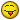##### Share on other sitesJust my 2 cents...

```
(setq Lst
'((-4 (0 (269.157 85.9418 0.0)) (0 (272.343 85.9418 0.0)) (0 (272.343 59.9463 0.0)) (0 (269.157 59.9463 0.0)))
(-3 (0 (273.021 84.9263 0.0)) (0 (274.851 84.9263 0.0)) (0 (274.851 83.3016 0.0)) (0 (273.021 83.3016 0.0)))
(-2 (0 (275.258 65.4297 0.0)) (0 (279.528 65.4297 0.0)) (0 (279.528 60.6233 0.0)) (0 (275.258 60.6233 0.0)))
(-1 (0 (280.206 72.2671 0.0)) (0 (283.596 72.2671 0.0)) (0 (283.596 69.5592 0.0)) (0 (280.206 69.5592 0.0)))
)
)

```

```
(foreach x Lst
(foreach y x
(if (listp y)
(setq testlist (cons (cadr y) testlist))
)
)
)

```

```
((280.206 69.5592 0.0) (283.596 69.5592 0.0) (283.596 72.2671 0.0) (280.206 72.2671 0.0) (275.258 60.6233 0.0) (279.528 60.6233 0.0) (279.528 65.4297 0.0) (275.258 65.4297 0.0) (273.021 83.3016 0.0) (274.851 83.3016 0.0) (274.851 84.9263 0.0) (273.021 84.9263 0.0) (269.157 59.9463 0.0) (272.343 59.9463 0.0) (272.343 85.9418 0.0) (269.157 85.9418 0.0))

```

## Join the conversation

You can post now and register later. If you have an account, sign in now to post with your account.
Note: Your post will require moderator approval before it will be visible.×   Pasted as rich text.   Paste as plain text instead

Only 75 emoji are allowed.